General Discussion General discussion about all things car audio, from pioneer, orion, alpine and eclipse.

Volts, amperes, hhhhhhh (long exhale). Help?

500 Watt CAFz'r

Join Date: Jan 2006
Posts: 607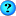Volts, amperes, hhhhhhh (long exhale). Help?

Haha, ya, this stuff sucks. I have been wondering around and reading a bunch on the bcae1 website and have learned a bunch of stuff but I am still really really lost. First I should get this question out of the way:

In the equation P=E^2/R what does "E" stand for??

Basically I am trying to learn how to calculate the true watts rms that my Ultimate T3 1000D amp can put out. I know its alot (imo) but I want to be exact so that I don't turn my gains up higher than the amp can cleanly put out and blow my woofer (know what I mean?).

I read on bcae1 that "continous rms power" is the watts that the amp will put out with out a doubt. "rms power" is the watts that the amp can put out but this rating may be for only a fraction of a second. And of course "peak" is, well i forgot how it was defined but ya everyone knows. SO! with that said how will I find out what my amp can do for burping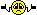??? I know I have 1200Watts at 2 ohm but I am sure this amp will do more than that. I have been given a few ways to semi-accuratly calculate the watts but I am wondering if a really accurate way exists???

The rules of the test that I want to do will be at 12v at a 2 ohm load. (I haven't seen the specs of this amp at 12v so ya, that is why I am trying to find out).

Thanks a bunch guys.1000 Watt CAFz'r

Join Date: Apr 2006
Posts: 1,029
BEEEEEEEEEEEEHOLDDDDDDDDDDDDDDDDDDDDDDDDDDDDDDDD.. ...

Nice and dramatic....

I notice that depending on which link you follow at the ultimate site, the amp is rated at 1200 @ 2 ohms (if you go support, then manuals and the T3 amplifier series PDF), but if you go to products and find the T31000D and check it the link to its PDF manual, it gives the 12.5V (2000RMS) rating and the 13.8 (2700RMS) as well. http://www.ultimate-sound.com/templa...chSpec1000.pdf500 Watt CAFz'r

Join Date: Jan 2006
Posts: 607
Ohhh ya, had ta be dramatic cuz, well I don't know why but I thought it was cool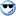. And yup I noticed all those ratings and stuff but it never gives a rating at 12v (the voltage that my battery puts out when the car is off) so I needta figure it out.1000 Watt CAFz'r

Join Date: Apr 2006
Posts: 1,029
Hmmm... well according to my formulas and schematics, in order to figure out what 12V would be, if given 12.5V, you need to times that number by 17, take the square root of that answer, add 186, divide that by the number of wheels your vehicle has, double that, then multiply by ten.

If the amp is rated at 2000W at 12.5V, that'd be:

2000 x 17 = 34,000
sqrt 34,000 = 184.39
184.39 + 186 = 370.39
370.39 / 4 (assuming your vehicle has four wheels) =
92.60 x 2 = 185.20
185.20 x 10 = 1852 Watts

So there you go, you should put out 1852 Watts at 12 volts500 Watt CAFz'r

Join Date: Jan 2006
Posts: 607
Hhhmmmmm, AWESOME. Haha, thanx for the help dude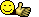.1000 Watt CAFz'r

Join Date: Apr 2006
Posts: 1,029
Ah crap double posted

Last edited by worldind; 09-03-2006 at 11:10 AM.1000 Watt CAFz'r

Join Date: Apr 2006
Posts: 1,029
On a more serious note, I suppose E would stand for electromotive force, or energy...

From what Ive read over on the termpro forum, to find the actual power I believe youll need a voltmeter that measures rms, (not peak) and an ammeter... during a burp, measure the voltage on the speaker leads and clamp the ammeter on one of the leads... then multiply these two figures V*I for power.

They also recommend that if your meter reads peak voltage, then multiply the voltage by .707 before using the last equation.

Correct me if Im wrong...

And this is assuming you want to actually physically test your stuff instead of attempting to solve it with just numbers.500 Watt CAFz'r

Join Date: Jan 2006
Posts: 607
Originally Posted by worldind

And this is assuming you want to actually physically test your stuff instead of attempting to solve it with just numbers.
thats wut I am goin for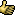. I think I will be goin and getting a new multi meter. Thnx.50 Watt CAFz'r

Join Date: Jul 2006
Posts: 56
The E is voltage in that equation. Power = volts^2/resistance. The E doesnt stand for energy, energy is the same as power. It is an electrical force/presure, but thats voltage.

amps = volts/ohms
power = volts * amps

then power = volts^2/ohms50 Watt CAFz'r

Join Date: Jul 2006
Posts: 56
Oh and about reading a peak voltage and multiplying my .707. Thats because most voltmeters dont measure "true rms" like the expensive ones do. rms stands for root mean square, so its kinda like a weighted average power. So given that your playing a pure sine wave, taking the peak and multiplying by .707 (actually square root of 2) will give you a theoretical true rms reading. But that only works for a sine wave, dont use it for playing music.Show Printable VersionEmail this Page